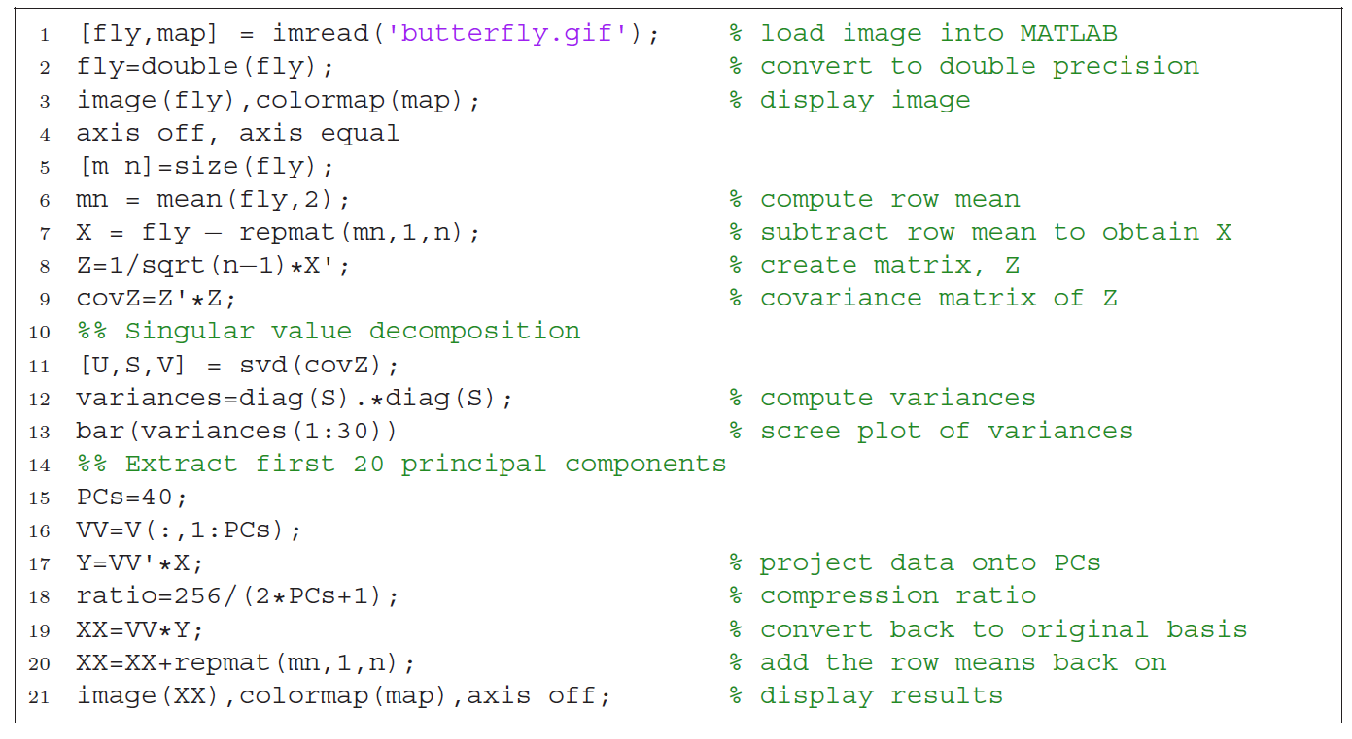# Complete and Simple PCA SVD Tutorial Note

Ref:

1.   http://setosa.io/ev/principal-component-analysis/
2. https://matthew-brett.github.io/teaching/pca_introduction.html
3. https://blog.statsbot.co/singular-value-decomposition-tutorial-52c695315254
4. http://www.bluebit.gr/matrix-calculator/calculate.aspx

PCA is the major method to reduce features/variables before you train your data in the machine learning. It uses the top K most variance transformed features to represent the original N features (assume N>>K).

For example, we have food consumption of 17 types of food in grams per person per week for every country in the UK.Maybe even after you view the above table for 5 minutes, you are hardly to get some patterns.

But if you use PCA to extract the first(principle) variance transformed feature as below, you may see some pattern that “N Ireland” is big different than other 3 countries in UK. Once we go back and look at the data in the table, this makes sense: the Northern Irish eat way more grams of fresh potatoes and way fewer of fresh fruits, cheese, fish and alcoholic drinks.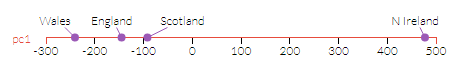Of course, you can further to use the top 2 transformed features to draw a x-y diagram as below.Or, you can only draw the pc1, pc2, pc3 data accordingly. You will find that PC1 has the most variation, the vertical axis PC2 the second-most, etc.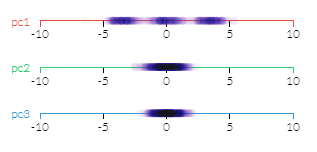Yes, PCA is not just used for machine learning. It is used for data analysis as one of the major statistics approach.  It can help you to be easy find out the pattern behind the data, even there are 10s or 100s features/variables.  As to machine learning emerging, it is used to reduce the feature dimensions when you train your data. Magically, you may only use 5 features to represent 100 original features.

Interesting? Let’s start!

1.  The original data can be treated as Vector.

Do not worry, just little math.  In the UK food consumption example above, each country can be treated as one vector with 17 dimensions. Below are the data for UK countries.

England(375,57,245,1472,105,54,193,147,1102,720,253,685,488,198,360,1374,156)

N Ireland(135,47,267,1494,66,41,209,93,674,1033,143,586,355,187,334,1506,139)

Scotland(458,53,242,1462,103,62,184,122,957,566,171,750,418,220,337,1572,147)

Wales(475,73,227,1582,103,64,235,160,1137,874,265,803,570,203,365,1256,175)

Then how can we get the most variation transformed feature? In statistics. The variation in one feature is to measure how difference of sample data in this feature.  To get variation, we need to standardized data for each feature x with formula (x-avg(x))/std(x). Here is the standardized data:

England(0.090930643,-0.044961799,-0.015151515,-0.557999447,0.570082574,-0.11977408,-0.547699747,0.558227286,0.638613269,-0.38977691,0.74764998,-0.22477362,0.327128997,-0.291214103,0.697563742,-0.376921203,0.113060199)

N Ireland(-1.440532816,-0.944197773,1.318181818,-0.155508043,-1.498123973,-1.365424508,0.167663188,-1.268698378,-1.393553862,1.169330729,-1.079938861,-1.284420687,-1.111157171,-1.092052886,-0.951223285,0.561825944,-0.985238881)

Scotland(0.620561756,-0.404656188,-0.196969697,-0.740950085,0.4640207,0.64678003,-0.950091397,-0.287571632,-0.049854567,-1.156877792,-0.614734428,0.470954252,-0.429863723,1.310463463,-0.760978628,1.031199518,-0.468392255)

Wales(0.729040418,1.39381576,-1.106060606,1.454457575,0.4640207,0.838418557,1.330127956,0.998042724,0.80479516,0.377323973,0.947023309,1.038240055,1.213891898,0.072803526,1.01463817,-1.216104259,1.340570936)

1.  From Data Vector to Features Covariance Matrix.

We use X(4,17) to represent all those data.  It will be easy to know that XT*X will be the Features co-variance of X times (n-1), as variance and covariance formula shown below.

.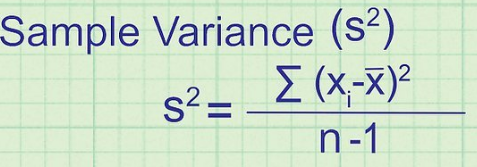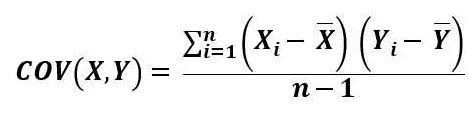This is easy to explain as below XT*X formula. The first matrix has 3 rows which are data of one Feature(such as Fish consumption); each column is one sample data (such as Wales country). As X=(X1,X2,X3) already standarized, diagnal datawill be the Variance of one Feature X1 times (n-1).is the covariance between features of X1 and X2, times (n-1).So, XT*X will be the co-variance of X times (n-1).

The Feature covariance matrix will show the level of data difference for each feature. Some may show big difference, some may show slight.

Extremely, if a feature of all sample data are same without any difference, the feature is useless for you to make distinguish of your sample data. For example, 5 students got all A in their transcript, how can a college admission officer to select the best one to admit?

So, most of time, we would like to find out the most variance feature(s) in the sample data. Further, the most variance feature maybe not the original features from sample data. It maybe the functions of those original features.

Let’s make an example. Suggest you have three points in a plane coordinates (X,Y).

1a) Sample 1: A(2, 2), B(2,3), C(2,5)  (below left diagram): only care sub of original features to represent sample data.

It will be easy to know that the Y feature is most variance. And variance in Y covers all variance in the sample data, as variance in X is zero.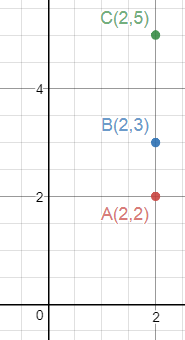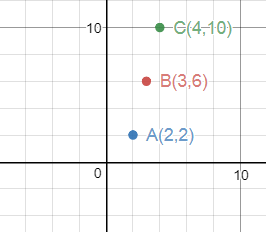1b) Sample 1: A(2, 2), B(3,6), C(4,10)  ((above right diagram): using one projected vector as new man-made features to represent sample data

It will be easy to know that the Y feature is still most variance.  However, if you only select Y feature to represent the variance of all the 3 sample data, you will lost the variance in X feature and the covariance between X,Y.

Below is the covariance calculation for the two examples.

In example 1a, the Y features variance will be the total of variance and covariance, as all are 0 except Y variance.

In example 1b, the Y features variance is 8, but the the total of variance and covariance is equal to 2+8+4=14.  So, Y feature does not cover all covariance of the 3 sample data. It only covers 8/14=57% variance.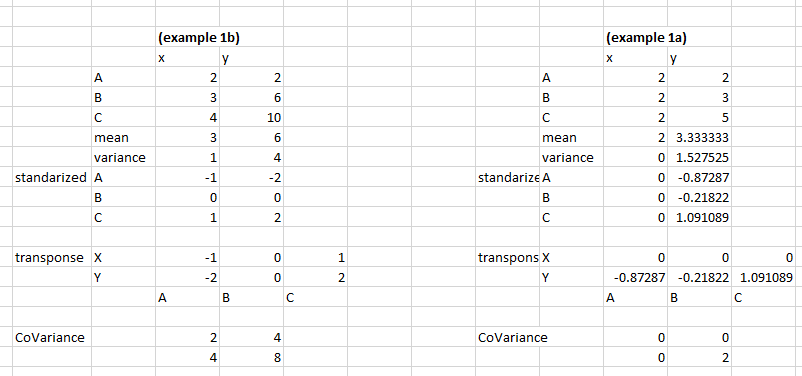So, for sample 1b, is it any better man-made feature that cover variance more than Y feature?

It is easy to know that the 3 points are in line Y=4X-6, but after standardized, they will set in line of Y=2X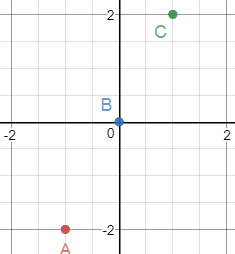Let’s ignore the covariance between X,Y. We only care for the variance of X and Y accordingly.

If we sum the variance X and variance Y in example 1b, you will get 8+2=10.

What does 10 means in the above plane coordinate diagram? You will find out that it is the sum of square length of each point to origin.  (10=5+0+5)

OA^2=1^2+2^2=5  ;    OB^2=0      ;   OC^2=1^2+2^2=5

So, we may want to find a direction (vector, or line) that maximum the sum of square length of each point that projected in the direction.  As we known, the line is Y=2X. We can set the direction vector as L(1,2), to make it calculate easy, we use unit direction vector as L, So after projected into the direction vector L, the length of vector A(-1,-2), B(0,0), C(1,2) will be the dot product with vector L accordingly, which areaccordingly. So, the sum of square length is equal to 10. (). After you project, the 3 sample vectors will become A:; B: (0,0);  C:=. Now they are all in the direction of (1,2).  Also, the new projected vectors cover all variance(total=10) from the original 3 sample data A(2, 2), B(3,6), C(4,10) .

That means we can definitely using the new projected vectors to represent the original three sample data, in terms of total variance.  As the 3 new projected vectors are in the same direction, we can rewrite them as A(), B(0), C(). That means we only use one dimension to represent the 3 points now.

If the first projected points can’t cover total variance, we need to the 2nd project vector to cover the remaining variance, and if 2nd vector still not able to cover all variance, we will use 3rd, 4th, etc.  Let’s see one more example below:

1c) Sample 1: A(2, 2), B(3,6), C(4,12)  ((above right diagram): using two or more projected vectors as new man-made features to represent sample data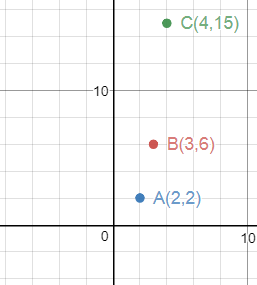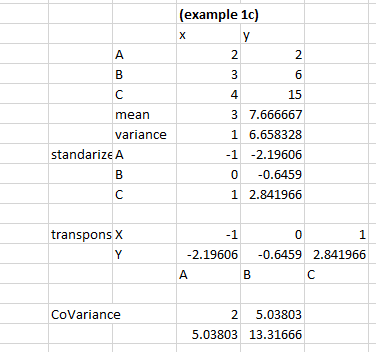Now the total variance is equal to 2+13.3=15.3. (Ignore the covariance of X,Y, which is equal to 5.038). Now which projected vector/direction for the 3 sample will contain the maximum variance?

Let’s try the projected vector Lwhich used in example 1b first.  Now the standardized A,B,C vectors will be as below:

A(-1,-2.196); B(0,-0.645); C(1,2.842)

After projected to vector L, the standardized A,B,C vectors will project to L as below. Note, we only write the length of each project as the direction will be as same as.

By using the vector project formula below, we can easily get the length after projection of 3 A,B,C Vectors. A,B,C Project lengths are 2.411, 0.576,2.989 accordingly.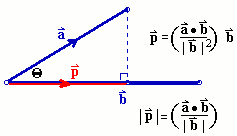So, the total variance in the projected to direction Lis equal to sum of square length= 2.411^2+ 0.576^2+2.989^2=15.0788. The variance is less than the original variance of 15.3.  The missing variance is the sum of square of length of one edge of right triangles. In the diagram below, we project A,B,C to the Line L (which is y=2x). The missing variance=sum(CC’^2+BB’^2+AA’^2).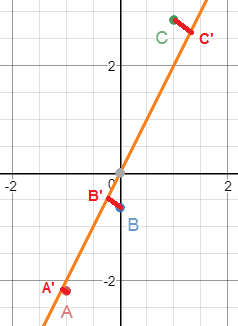Why? After projected, we can calculate the residual of A,B,C vectors. That is easy. According to vector operation, we knew that AA’=OA-OA’,  BB’=OB-OB’, CC’=OC-OC’.

So, if we want to cover those missing variance of the vectors, we need to have another direction/vector L’ to get the max variance of residual vectors. As all those residual vectors has same directions (all are orthogonal to the line L, y=2x), another projection L’ will be orthogonal to L which will get the max residual variance (as it cover all residual variance).

We may say, by project A,B,C into orthogonal L and L’ vectors, we will redeploy the variances of original vectors into two orthogonal directions. Actually, we can treat the two lines as the new coordinate with x=L and y=L’. The sum of square of new coordinate (x,y) of A,B,C in the new coordinate will be the sum of the original variance in old coordinate. This is easily proof by the Pythagorean Theorem.  For example, square Length of original OC is equal to square of length OC’+CC’, while (OC’,CC’) is the coordinate of C in new coordinate of L and L’.

Ok, you can see we can always use orthogonal L, L’ to re-assign the original variance into two parts. In the example 1c, we are just randomly use the first vector L (y=2x).  Actually, you can do any pairs of orthogonal lines to re-assign the original variance into two parts.

So, if we want to max the variance in the first vector, how can we do that? That is the basic motive of PCA.

1d) Sample 1: A(2, 2), B(3,6), C(4,12) : maximize the variance in the first projected vectors

We can treat projection direction L=(0.447,0,894) as a projection angle in a unit circle. You will have angle from 0 to 180 degree (as 180 to 360 will the same line as 0 to 180). For example. The blue line. So, you can write a small program to find out the minimum residual after projection into the direction of the angle.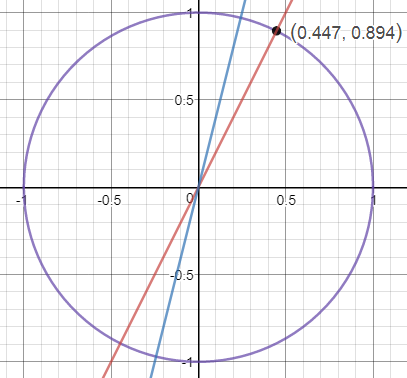Copied from https://matthew-brett.github.io/teaching/pca_introduction.html

#gnote: my example

import numpy as np

import matplotlib.pyplot as plt

def line_projection(u, X):

“”” Return columns of X projected onto line defined by u

“””

u = u.reshape(1, 2)  # A row vector

c_values = u.dot(X)  # c values for scaling u

projected = u.T.dot(c_values)

return projected

def line_remaining(u, X):

“”” Return vectors remaining after removing cols of X projected onto u

“””

projected = line_projection(u, X)

remaining = X – projected

return remaining

angles = np.linspace(0, np.pi, 10000)

x = np.cos(angles)

y = np.sin(angles)

u_vectors = np.vstack((x, y))

u_vectors.shape

remaining_ss = []

Xg = np.asarray([[-1,0,1],[-2.196,-0.645,2.842]])

for u in u_vectors.T: # iterate over columns

remaining = line_remaining(u, Xg)

remaining_ss.append(np.sum(remaining ** 2))

plt.plot(angles, remaining_ss)

plt.xlabel(‘Angle of unit vector’)

plt.ylabel(‘Remaining sum of squares’)

min_i = np.argmin(remaining_ss)

angle_best = angles[min_i]

print(angle_best)

x1 = np.cos(angle_best)

y1 = np.sin(angle_best)

first_vector = np.vstack((x1, y1))

print(first_vector)

print(remaining_ss[min_i])

→ result:

1.20712060957

[[ 0.355712]

[ 0.934596]]

0.0820150803757

Ok, from the result, we can see that the best first projected vector is (0.355, 0.934), and the variance it covers is equal to 15.3-0.082=15.218, which is best than previous random direction L=(0.447,0,894)  with covered variance of 15.0788.  You can draw it together as below diagram. The orange line is the old direction, the black line is the new direction. You can see the new direction black line is closer with B and C than orange line.

[Image_Link]https://lh6.googleusercontent.com/qj2uObpzb

Yes. That is PCA.  Max the variance in the first vector, then 2nd vector, then 3rd, until no more residual variance.

Now, let’s make another example to compare the variance of two projected vectors.

 (example 1b) x y A 2 2 B 3 6 C 4 10 mean 3 6 variance 1 4 standarized A -1 -2 B 0 0 C 1 2 transponse X -1 0 1 Y -2 0 2 A B C CoVariance 2 4 4 8

 (example 1c) x y A 2 2 B 3 6 C 4 15 mean 3 7.666667 variance 1 6.658328 standarized A -1 -2.19606 B 0 -0.6459 C 1 2.841966 transponse X -1 0 1 Y -2.19606 -0.6459 2.841966 A B C CoVariance 2 5.03803 5.03803 13.31666

1.  how to get a max transfer vector:The two.  This can be easily found out via graph.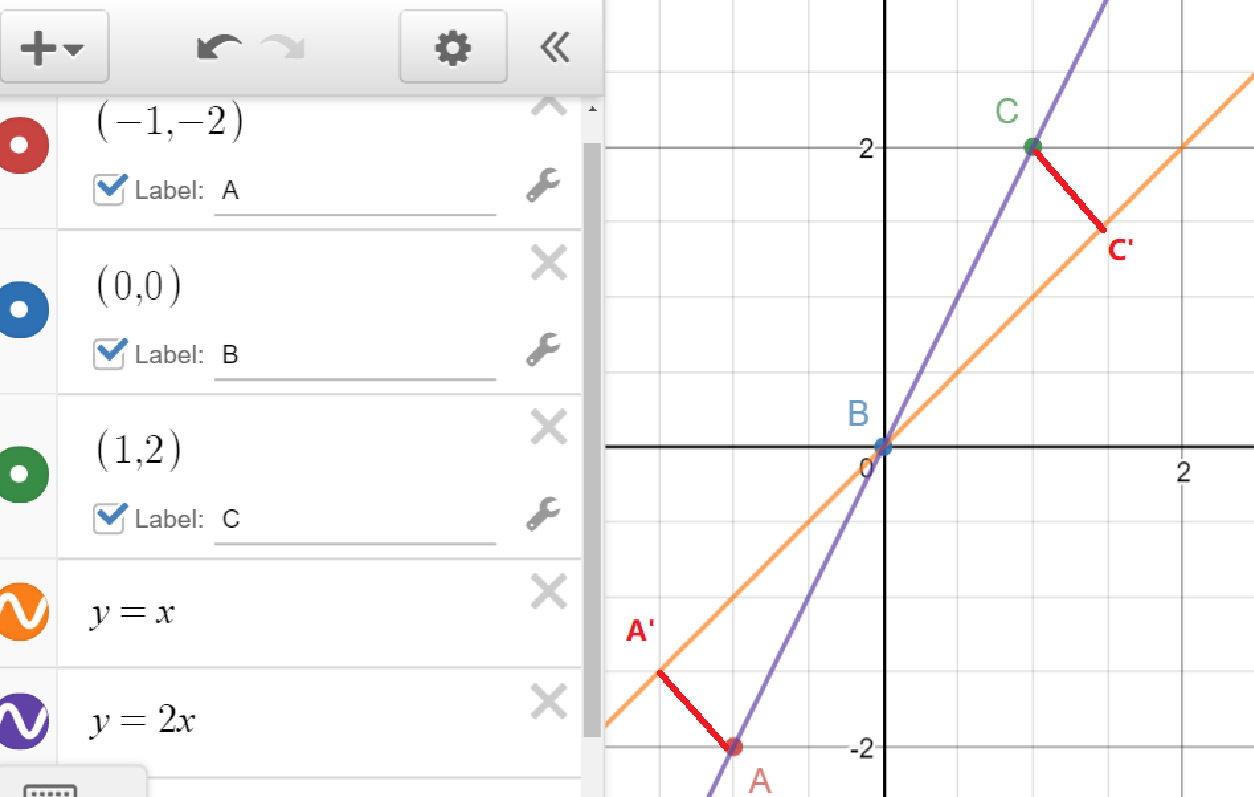It is obviously that A,B,C has bigger variance than A’,B, C’ in its vector(line) direction accordingly, as in right triangle, the hypotenuse is the biggest edge. so, AB>A’B, BC>BC’.

Now, How can we find out the max?

2.  SVD: Most effective way to calculate the principal vectors of PCA.

Now, you get the idea of PCA. We call the projected first, second, 3rd or more as the principal components of the original sample data.  For example, you may select top 10 principal components/vectors to represent your 100 dimensions/features of original data. That will shrink the data space 90%, which increase your speed to data training and analyze. Further, if you only use two or three principal components, you can draw it in 2d or 3d to help you visualize the data analysis.  (we will use the principal components as the same meaning of vectors below.)

But, in the first session, it is a little bit tedious to get those vectors.  Luckily, we will use Matrix SVD (single value decomposition) to get those vectors. When using the SVD, we decompose our sample data X into three matrix, orthogonal eigenvector matrix, diagonal eigenvalue matrix of variance and the 3rd orthogonal matrix. Here is the example. The eigenvector matrix is a set of the PCA principle vectors, while the eigenvalue matrix includes the corresponding variance covered by the vectors in the diagonal cells.

(note: Eigenvalue decomposition is different than the SVD decomposition. Eigenvalue of a matrix A in Eigenvalue decomposition is same as SVD eigenvalue diagonal matrix when the matrix A is symmetric and all value in SVD eigenvalue diagonal matrix are positive.   Here, variance matrix are always symmetric-and-positive-definite, which will have positive eigenvalue)

https://stats.stackexchange.com/questions/52976/is-a-sample-covariance-matrix-always-symmetric-and-positive-definite

)

In 2D geometry, if you multiple a point (that is vector) in the coordinate with the matrix, you can image the point are rotate twice in an ellipse with eigenvalues as long axis and short axis. You can treat the first and the 3rd orthogonal matrix as first and second rotation angles of the point in the ellipse.So, the first principle vector will be vector L(-0.356,-0.935) with corresponding covered variance of 3.903. This looks different than the original one L(0.356,0.935) from python result. Actually, the two vectors are the same projection lines with different direction only. It will have the same result for sample data to project to the two vectors.

That makes life easier to get the principle vector via SVD method. But wait a minute. Why the SVD produced the variance of 3.903 instead of python variance result of 15.218?

The variance difference is caused by the matrix we used in python and SVD. In the python, we use thewhile X in SVD.  Here is the proof.This represents that when we handle the SVD with X, the variance it covered in the projected vector will have square root of that produced with.  You can verify it with the example above that 3.903*3.903=15.21.
You may be curious why it works? Don’t worry, let’s prove it with strict math. It worths to understand the theory behind.

3.  Why SVD works to calculate the principal vectors of PCA?4.  SVD for real example

Ok, let’s back to the original example in the opening of the note, to analyze food consumption of 17 types of foods for 4 countries in Britain.  Below is the sample data X after centralized (i.e. minus mean for each dimension).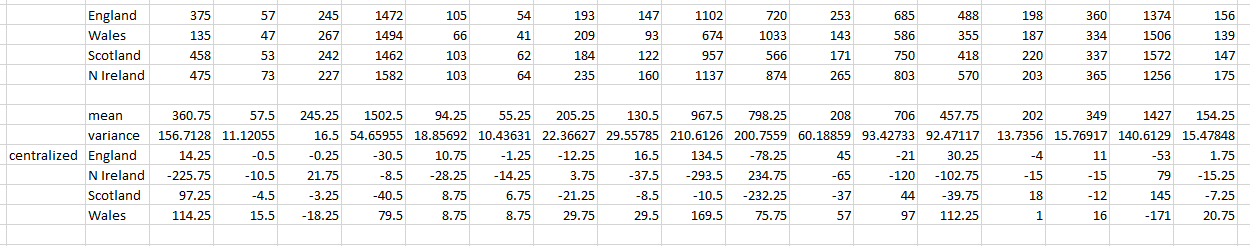With SVD, the variance of X can be decomposed as below: (thanks to the online matrix tool http://www.bluebit.gr/matrix-calculator/calculate.aspx)

*a) Get variance of X: X’*X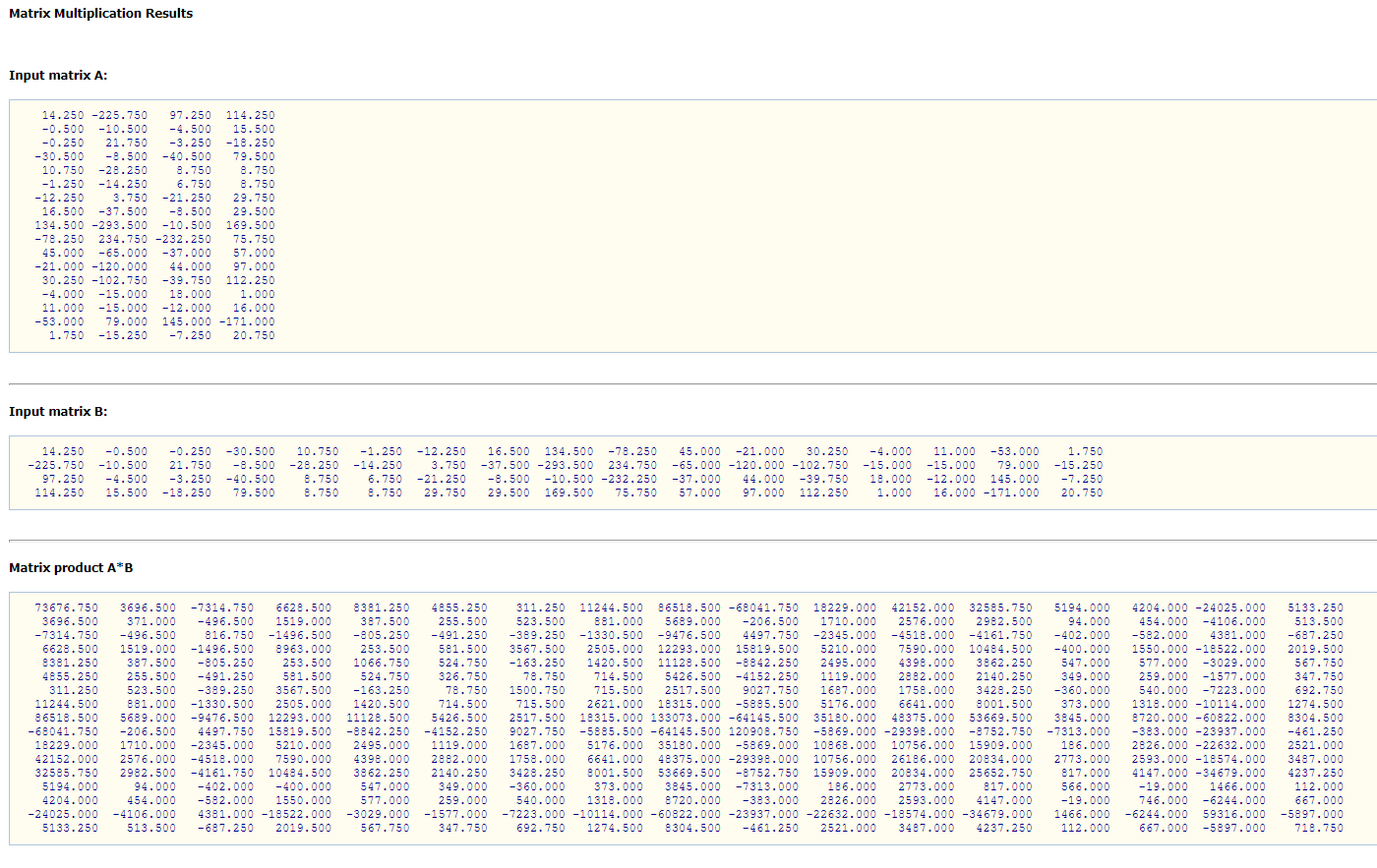*b) SVD Decompose for variance of X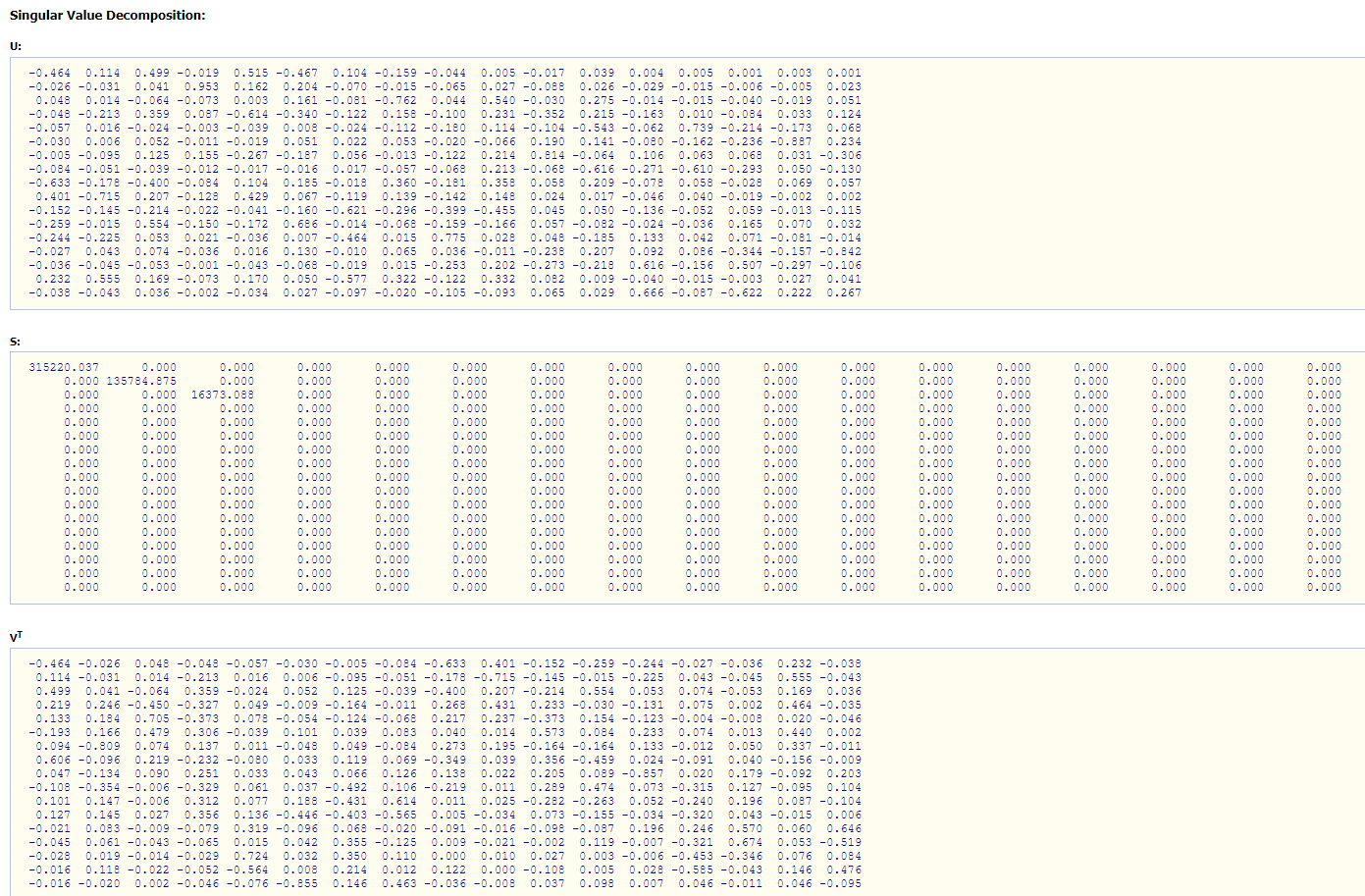*c) X data projection to PCA vectors (i.e. U matrix in SVD result): X*U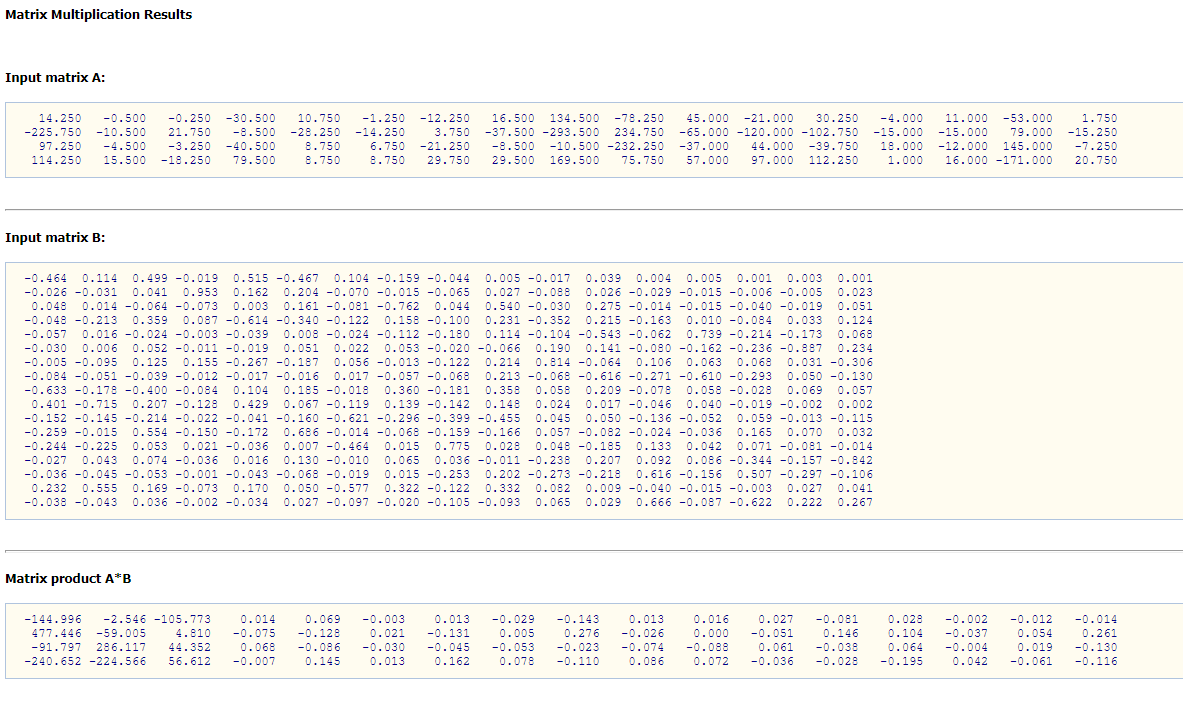The X data projected to the top 1 and 2 PCA vectors is shown in the column 1 and column 2 in the diagram above. According to the diagram below, you can see that “N Ireland” is much different than other 3 countries.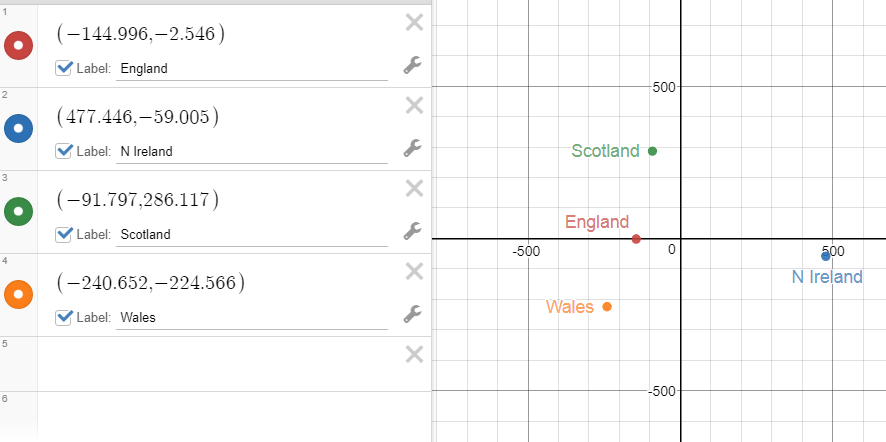The above diagram looks different than the previous diagram shown in the start of the note. This is caused by different ways of pre-processing of data. In my example here, I just centralized data, i.e. minus mean for each dimensions.

1. Finally, we may talk about data recovery from top k principle PCA components.

When we use the variance to do the SVD. The U and V matrix are same as variance matrix are symmetric.

As we mentioned before, you can project your data into the top k principal components with formula. Hereis to select k columns from original. After that, you can useto get the recovery data from. Of course, if the k is not the full set of columns of,will be different than original.

You may think Y is the compression data of X. It is useful in the image compression.  Here is the matlab code for the procedure. It is easy to read and try. (Left side is the original test grey 512×512 image, right side the image after recovery from compression with ratio 6.3:1.)